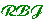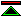Math by Proof
What is it, and why should we?

Formalised mathematics is distinguished from informal mathematics by three features:

• Machine processable languages with precisely defined semantics in which mathematical propositions are expressed.
• Machine checkable reliable criteria for demonstrating the truth of mathematical propositions.
• Machine checkable criteria permitting the introduction of new meaningful formal vocabulary without compromising the consistency of the logical system.

These methods are potentially applicable not just in those areas of mathematics where discovering and proving new mathematical results is the central purpose, but in all aspects of mathematics whether or not they are normally associated with proof.

There is nothing in the stated criteria for formalisation which excludes efficient arithmetic computation and algebraic manipulations from the domain of formalised mathematics.

Among the benefits of doing mathematics by formal proof are:

• Precision
Formal mathematics is conducted with absolute precision. Intelligent informal assessment of the magnitude of errors arising from floating point rounding errors is not required.
This contributes to:
• Trust
Tools supporting formal mathematics when well designed and implemented are highly trustworthy, establishing the truth of mathematical propositions with almost absolute certainty.
• Functionality
The combination of precision and trust makes possible new kinds of functionality. The fact that the correctness of alleged solutions to mathematical problems can be reliably checked means that otherwise unreliable efficient problem solving algorithms can be safely applied.©created 1996/4/8 modified 1996/10/5